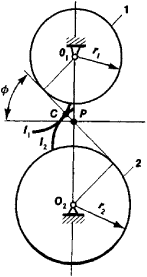# Involute Gearing

The following article is from The Great Soviet Encyclopedia (1979). It might be outdated or ideologically biased.

## Involute Gearing

a gear tooth system in which the tooth contours are described by the involute of a circle, known as the base circle.

Figure 1 shows the involute tooth system of a gear train. The tooth contour of gear wheel 1 is described by the involute I1 of the base circle with the radius r1 and the tooth contour of gear wheel 2 is described by the involute I2 of the base circle with the radius r2. The involutes I1 and I2 are tangent at the point C. According to the conditions for the construction of an involute, the normal to involute I1 at the point C must be tangent to the base circle with the radius r1 and the normal to involute I2 must be tangent to the base circle with the radius r2. From this it follows that in an involute tooth system the common normal to the contiguous contours of the teeth always coincides with the common tangentFigure 1. Involute gearing: (1) and (2) gear wheels

to the base circles. This tangent is known as the line of action because the point of contact between the teeth moves along the line as the gear wheels move. The point P where the line of action intersects the line of centers O1O2 is called the pitch point, and the angle made by the line of action with the perpendicular to the line of centers is the pressure angle ϕ. The gear ratio mG, that is, the ratio of the angular velocities of gear wheels 1 and 2, is given for involute gearing by mG = O2P/O1P = r2/r1 It is therefore evident that with involute gearing a change in the center distance does not affect the gear ratio but only the pressure angle. Involute gears are the most common type in use.

N. I. LEVITSKII